Updating search results...

# 13 Results

View
Selected filters:
• MCCRS.Math.Content.7.SP.B.3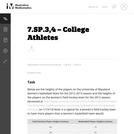Unrestricted Use
CC BY
Rating
0.0 stars

n this task, students are able to conjecture about the differences in the two groups from a strictly visual perspective and then support their comparisons with appropriate measures of center and variability. This will reinforce that much can be gleaned simply from visual comparison of appropraite graphs, particularly those of similar scale. Students are also encouraged to consider how certain measurements and observation values from one group compare in the context of the other group.

Subject:
Mathematics
Statistics and Probability
Material Type:
Activity/Lab
Provider:
Illustrative Mathematics
Provider Set:
Illustrative Mathematics
Author:
Illustrative Mathematics
02/24/2013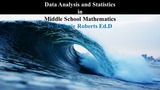Unrestricted Use
CC BY
Rating
0.0 stars

This supplemental resource provides problems and activities related to Data Analysis and Statistics in Middle School Mathematics.

Subject:
Mathematics
Material Type:
Activity/Lab
Author:
Twianie Roberts Ed.D
09/25/2022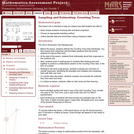Only Sharing Permitted
CC BY-NC-ND
Rating
0.0 stars

This lesson unit is intended to help you assess how well students are able to: solve simple problems involving ratio and direct proportion; choose an appropriate sampling method; and collect discrete data and record them using a frequency table.

Subject:
Education
Geometry
Mathematics
Measurement and Data
Ratios and Proportions
Material Type:
Assessment
Lecture Notes
Lesson Plan
Teaching/Learning Strategy
Provider:
Shell Center for Mathematical Education
Provider Set:
Mathematics Assessment Project (MAP)
Author:
http://map.mathshell.org/
04/26/2013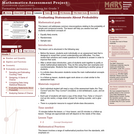Only Sharing Permitted
CC BY-NC-ND
Rating
0.0 stars

This lesson unit addresses common misconceptions relating to probability of simple and compound events. The lesson will help you assess how well students understand concepts of: Equally likely events; randomness; and sample sizes.

Subject:
Mathematics
Statistics and Probability
Material Type:
Assessment
Lesson Plan
Provider:
Shell Center for Mathematical Education
Provider Set:
Mathematics Assessment Project (MAP)
04/26/2013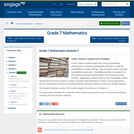Conditional Remix & Share Permitted
CC BY-NC-SA
Rating
0.0 stars

In this module, students begin their study of probability, learning how to interpret probabilities and how to compute probabilities in simple settings.  They also learn how to estimate probabilities empirically.  Probability provides a foundation for the inferential reasoning developed in the second half of this module.  Additionally, students build on their knowledge of data distributions that they studied in Grade 6, compare data distributions of two or more populations, and are introduced to the idea of drawing informal inferences based on data from random samples.

Subject:
Mathematics
Statistics and Probability
Material Type:
Module
Provider:
New York State Education Department
Provider Set:
EngageNY
01/06/2014Conditional Remix & Share Permitted
CC BY-NC
Rating
0.0 stars

Four full-year digital course, built from the ground up and fully-aligned to the Common Core State Standards, for 7th grade Mathematics. Created using research-based approaches to teaching and learning, the Open Access Common Core Course for Mathematics&nbsp;is designed with student-centered learning in mind, including activities for students to develop valuable 21st century skills and academic mindset.

Subject:
Mathematics
Material Type:
Full Course
Provider:
Pearson
10/06/2016Conditional Remix & Share Permitted
CC BY-NC
Rating
0.0 stars

Samples and ProbabilityType of Unit: ConceptualPrior KnowledgeStudents should be able to:Understand the concept of a ratio.Write ratios as percents.Describe data using measures of center.Display and interpret data in dot plots, histograms, and box plots.Lesson FlowStudents begin to think about probability by considering the relative likelihood of familiar events on the continuum between impossible and certain. Students begin to formalize this understanding of probability. They are introduced to the concept of probability as a measure of likelihood, and how to calculate probability of equally likely events using a ratio. The terms (impossible, certain, etc.) are given numerical values. Next, students compare expected results to actual results by calculating the probability of an event and conducting an experiment. Students explore the probability of outcomes that are not equally likely. They collect data to estimate the experimental probabilities. They use ratio and proportion to predict results for a large number of trials. Students learn about compound events. They use tree diagrams, tables, and systematic lists as tools to find the sample space. They determine the theoretical probability of first independent, and then dependent events. In Lesson 10 students identify a question to investigate for a unit project and submit a proposal. They then complete a Self Check. In Lesson 11, students review the results of the Self Check, solve a related problem, and take a Quiz.Students are introduced to the concept of sampling as a method of determining characteristics of a population. They consider how a sample can be random or biased, and think about methods for randomly sampling a population to ensure that it is representative. In Lesson 13, students collect and analyze data for their unit project. Students begin to apply their knowledge of statistics learned in sixth grade. They determine the typical class score from a sample of the population, and reason about the representativeness of the sample. Then, students begin to develop intuition about appropriate sample size by conducting an experiment. They compare different sample sizes, and decide whether increasing the sample size improves the results. In Lesson 16 and Lesson 17, students compare two data sets using any tools they wish. Students will be reminded of Mean Average Deviation (MAD), which will be a useful tool in this situation. Students complete another Self Check, review the results of their Self Check, and solve additional problems. The unit ends with three days for students to work on Gallery problems, possibly using one of the days to complete their project or get help on their project if needed, two days for students to present their unit projects to the class, and one day for the End of Unit Assessment.

Subject:
Mathematics
Statistics and Probability
Material Type:
Unit of Study
Provider:
PearsonConditional Remix & Share Permitted
CC BY-NC
Rating
0.0 stars

Students estimate the length of 20 seconds by starting an unseen timer and stopping it when they think 20 seconds has elapsed. They are shown the results and repeat the process two more times. The first and third times are recorded and compiled, producing two data sets to be compared. Students analyze the data to conclude whether or not their ability to estimate 20 seconds improves with practice.Key ConceptsMeasures of center and spreadLine plots, box plots, and histogramsMean absolute deviation (MAD)Goals and Learning ObjectivesApply knowledge of statistics to compare sets of data.Use measures of center and spread to analyze data.Decide which graph is appropriate for a given situation.

Subject:
Statistics and Probability
Material Type:
Lesson Plan
09/21/2015Conditional Remix & Share Permitted
CC BY-NC
Rating
0.0 stars

Students will apply their knowledge of statistics learned in sixth grade. They will determine the typical class score from a sample of the population, and reason about the representativeness of the sample.Students analyze test score data from a fictitious seventh grade class and make generalizations about district-wide results. They then compare the data to a second seventh grade class and reason about whether these are random samples. Students will review measures of center and spread as they find evidence to draw conclusions about the data.Key ConceptsSample size will be considered as it affects the conclusions of an analysis of a population.Students will review tools that they used in sixth grade to analyze data, such as measures of center and spread, and different types of graphs.Goals and Learning ObjectivesExplore sample size.Look at the effects of using a nonrandom sample.Review tools used to analyze data.

Subject:
Statistics and Probability
Material Type:
Lesson Plan
09/21/2015Conditional Remix & Share Permitted
CC BY-NC
Rating
0.0 stars

Students estimate the length of 50 seconds by starting an unseen timer and stopping it when they think 50 seconds has elapsed. The third attempt is recorded and compiled into a data set, which students then compare to the third attempt from the previous lesson when they estimated the length of 20 seconds. Students analyze the data to make conclusions about how well seventh grade students can estimate lengths of time.Students repeat the timing activity for 50 seconds, but only the third trial is recorded. The task today is to compare this set of data with the third trial for 20 seconds. Students will need to deal with the difference in the spread of data, as well as how to compare the data sets. Students will be reminded of Mean Absolute Deviation (MAD), which will be a useful tool in this situation.Key ConceptsStudents apply the tools learned in Unit 6.8:Measures of center and spreadMean absolute deviation (MAD)Goals and Learning ObjectivesApply knowledge of statistics to compare different sets of data.Use measures of center and spread to analyze data.

Subject:
Statistics and Probability
Material Type:
Lesson Plan
09/21/2015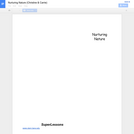Conditional Remix & Share Permitted
CC BY-NC
Rating
0.0 stars

Our project involves students learning about the values of a natural area in their community and producing a public service announcement and map to show its value and how it could be developed.

Subject:
Career and Technical Education
Ecology
Environmental Studies
Life Science
Material Type:
Activity/Lab
Lesson Plan
Provider:
Lane County STEM Hub
Provider Set:
Content in Context SuperLessons
09/29/2015Unrestricted Use
CC BY
Rating
0.0 stars

In this task, students are able to conjecture about the differences and similarities in the two groups from a strictly visual perspective and then support their comparisons with appropriate measures of center and variability. This will reinforce that much can be gleaned simply from visual comparison of appropriate graphs, particularly those of similar scale.

Subject:
Mathematics
Statistics and Probability
Material Type:
Activity/Lab
Provider:
Illustrative Mathematics
Provider Set:
Illustrative Mathematics
Author:
Illustrative Mathematics
02/24/2013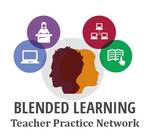Conditional Remix & Share Permitted
CC BY-NC
Rating
0.0 stars

Students will explore the overarching question, is the internet trustworthy?, while expanding their knowledge of statistics and comparing data sets. &nbsp;They will compare two data sets as well as determine the accuracy or bias of data representations shown on the internet. Students will then have the opportunity to apply their knowledge while creating their own visual representations for data they personally collected regarding the trustworthiness of the internet. &nbsp;The module concludes with a peer showcase and the post-assessment.

Subject:
Measurement and Data
Statistics and Probability
Material Type:
Lesson Plan
Author:
Blended Learning Teacher Practice Network